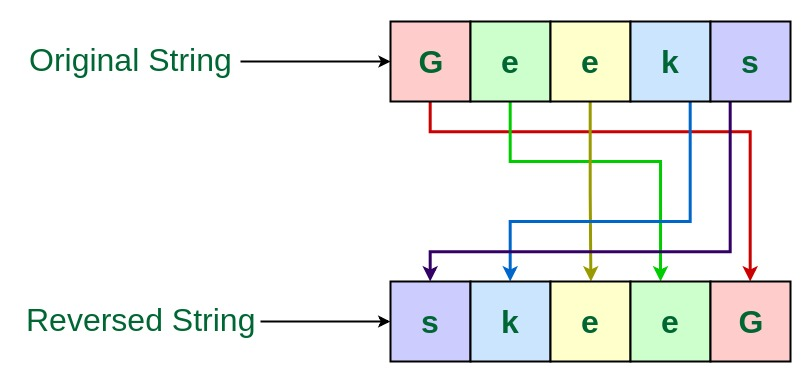# TCS Coding Practice Question | Reverse a String

Given a string, the task is to reverse this String using Command Line Arguments.

Examples:

```Input: Geeks
Output: skeeG

Input: GeeksForGeeks
Output: skeeGroFskeeG
```Approach 1: Using another String to store the reverse

• Since the string is entered as Command line Argument, there is no need for a dedicated input line
• Extract the input string from the command line argument
• Create a String to store the resultant reversed string, say reversedString
• Traverse through this String character by character using loop, in reverse order
• Now append each character into the resultant reversedString
• This is the required reversed string

Program:

## C

 `// C program to reverse a string ` `// using command line arguments ` ` `  `#include ` `#include ` `#include ` ` `  `// Function to reverse the String ` `char``* reverseString(``char` `input[]) ` `{ ` ` `  `    ``// Get the length of the string ` `    ``int` `length = ``strlen``(input); ` `    ``int` `i; ` ` `  `    ``// String to store the reverse ` `    ``char``* reversedString ` `        ``= (``char``*)``malloc``(length * ``sizeof``(``char``)); ` ` `  `    ``// Loop through the string ` `    ``// character by character in reverse order ` `    ``// and store it into the resultant string ` `    ``for` `(i = length - 1; i >= 0; i--) ` `        ``reversedString[length - 1 - i] = input[i]; ` ` `  `    ``// Return the reversed String ` `    ``return` `reversedString; ` `} ` ` `  `// Driver code ` `int` `main(``int` `argc, ``char``* argv[]) ` `{ ` `    ``// Check if the length of args array is 1 ` `    ``if` `(argc == 1) ` `        ``printf``(``"No command line arguments found.\n"``); ` `    ``else` `{ ` ` `  `        ``// Get the command line argument ` `        ``// and reverse it ` `        ``printf``(``"%s\n"``, reverseString(argv)); ` `    ``} ` `    ``return` `0; ` `} `

## Java

 `// Java program to reverse a string ` `// using command line arguments ` ` `  `class` `GFG { ` ` `  `    ``// Function to reverse the String ` `    ``public` `static` `String reverseString(String input) ` `    ``{ ` ` `  `        ``// String to store the reverse ` `        ``String reversedString = ``""``; ` ` `  `        ``// Loop through the string ` `        ``// character by character in reverse order ` `        ``// and store it into the resultant string ` `        ``for` `(``int` `i = input.length() - ``1``; i >= ``0``; i--) ` `            ``reversedString += input.charAt(i); ` ` `  `        ``// Return the reversed String ` `        ``return` `reversedString; ` `    ``} ` ` `  `    ``// Driver code ` `    ``public` `static` `void` `main(String[] args) ` `    ``{ ` ` `  `        ``// Check if length of args array is ` `        ``// greater than 0 ` `        ``if` `(args.length > ``0``) { ` ` `  `            ``// Get the command line argument ` `            ``// and reverse it ` `            ``System.out.println(reverseString(args[``0``])); ` `        ``} ` `        ``else` `            ``System.out.println(``"No command line "` `                               ``+ ``"arguments found."``); ` `    ``} ` `} `

Output:

Approach 2: Without using another String to store the reverse

• Since the string is entered as Command line Argument, there is no need for a dedicated input line
• Extract the input string from the command line argument
• Traverse through this String character by character using loop till half the length of the sting
• Swap the characters from one end with the characters from the other end
• This is the required reversed string

Program:

## C

 `// C program to reverse a string ` `// using command line arguments ` ` `  `#include ` `#include ` `#include ` ` `  `// Function to reverse a string ` `char``* reverseString(``char` `str[]) ` `{ ` ` `  `    ``int` `n = ``strlen``(str); ` `    ``int` `i; ` `    ``char` `temp; ` ` `  `    ``// Swap character starting from two ` `    ``// corners ` `    ``for` `(i = 0; i < n / 2; i++) { ` `        ``temp = str[i]; ` `        ``str[i] = str[n - i - 1]; ` `        ``str[n - i - 1] = temp; ` `    ``} ` ` `  `    ``return` `str; ` `} ` ` `  `// Driver code ` `int` `main(``int` `argc, ``char``* argv[]) ` `{ ` `    ``// Check if the length of args array is 1 ` `    ``if` `(argc == 1) ` `        ``printf``(``"No command line arguments found.\n"``); ` `    ``else` `{ ` ` `  `        ``// Get the command line argument ` `        ``// and reverse it ` `        ``reverseString(argv); ` ` `  `        ``// Print the reversed string ` `        ``printf``(``"%s\n"``, argv); ` `    ``} ` `    ``return` `0; ` `} `

## Java

 `// Java program to reverse a string ` `// using command line arguments ` ` `  `class` `GFG { ` ` `  `    ``// Function to reverse the String ` `    ``public` `static` `String reverseString(String str) ` `    ``{ ` ` `  `        ``int` `n = str.length(); ` `        ``char` `temp; ` ` `  `        ``// Swap character starting from two ` `        ``// corners ` `        ``for` `(``int` `i = ``0``; i < n / ``2``; i++) { ` `            ``str = str.substring(``0``, i) ` `                  ``+ str.charAt(n - i - ``1``) ` `                  ``+ str.substring(i + ``1``, n - i - ``1``) ` `                  ``+ str.charAt(i) ` `                  ``+ str.substring(n - i); ` `        ``} ` ` `  `        ``return` `str; ` `    ``} ` ` `  `    ``// Driver code ` `    ``public` `static` `void` `main(String[] args) ` `    ``{ ` ` `  `        ``// Check if length of args array is ` `        ``// greater than 0 ` `        ``if` `(args.length > ``0``) { ` ` `  `            ``// Get the command line argument ` `            ``// and reverse it ` `            ``System.out.println(reverseString(args[``0``])); ` `        ``} ` `        ``else` `            ``System.out.println(``"No command line "` `                               ``+ ``"arguments found."``); ` `    ``} ` `} `

Output:

Attention reader! Don’t stop learning now. Get hold of all the important DSA concepts with the DSA Self Paced Course at a student-friendly price and become industry ready.

My Personal Notes arrow_drop_upContent Manager at LambdaTest

If you like GeeksforGeeks and would like to contribute, you can also write an article using contribute.geeksforgeeks.org or mail your article to contribute@geeksforgeeks.org. See your article appearing on the GeeksforGeeks main page and help other Geeks.

Please Improve this article if you find anything incorrect by clicking on the "Improve Article" button below.

Article Tags :
Practice Tags :

4

Please write to us at contribute@geeksforgeeks.org to report any issue with the above content.